# Materials and Components – Section 3(Page 3)

Materials and Components – Section 3

21.  The dielectric constant of ferroelectric materials can be expressed by the equation
 A.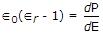B.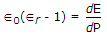C.D.22.  A liquid has N permanent dipoles μp per unit volume and has been subjected to dc field for a long time such that orientation polarization is P0. At t = 0, the field is suddenly removed, then polarization
 A. will remain constant at P0 B. will become zero at t = 0 C. will delay to zero with a time constant D. either b or c

23. Assertion (A): Orbital magnetic moment of an electron in an atom is of the order of 1 Bohr magneton.

Reason (R): 1 Bohr magneton = 9.27 x 10-24 A/m2.

 A. Both A and R are true and R is correct explanation of A B. Both A and R are true but R is not correct explanation of A C. A is true but R is false D. A is false but R is true

24.  As per Bohr’s postulate, only those circular orbits are stable for which the angular momentum is equal to an integer times.
 A. True B. False

Explanation:

It is a quantum condition postulated by Bohr.

25.  Inductors used at V.H.F. are made using
 A. air core B. cast iron cored C. sheet steel cored D. thin film technique

Explanation:

Thin film technique ensure low hysteresis and eddy current losses.

26.  If a capacitor C having selectivity factor Q is scaled down in all its dimensions by a factor K without any altering the dielectric material, the new capacitor will have capacitance and selectivity as
 A. C,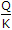B., Q C.,D. C, K

Explanation:

Since the dimension of plates is scaled down by K, the area decreases by a factor of K2, the distance between plates decreases by a factor of K.

Therefore, capacitance changes to.

The selectivity will not be affected.

27.  The hysteresis loss is
 A. proportional to frequency B. independent of frequency C. proportional to (frequency)2 D. proportional to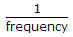28.  If m, v, r are the mass, velocity and radius of orbit of electron and h is Planck’s constant, quantum condition for electron’s orbit is
 A.B.C.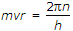D.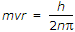Explanation:

For stable circular orbit angular momentum is equal to integer times.

29. Assertion (A): For a magnetic material M = (μr – 1) H, where M is magnetic dipole moment/m3, H is field intensity A/m and μr is relative permeability.

Reason (R): μr can have any value from 0 to hundreds of thousands.

 A. Both A and R are true and R is correct explanation of A B. Both A and R are true but R is not correct explanation of A C. A is true but R is false D. A is false but R is true

Explanation:

μr is always more than 1. It cannot be 0 or less than 1.

30.  As regards ∈0 and ∈r (absolute and relative permittivity)
 A. both ∈0 and ∈r can be interpreted in terms of atomic properties of medium B. only ∈r can be interpreted in terms of atomic properties of medium C. only ∈0 can be interpreted in terms of atomic properties D. ∈r has no physical significance